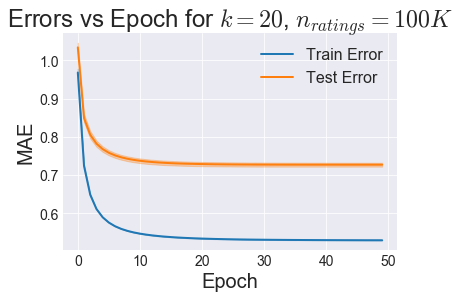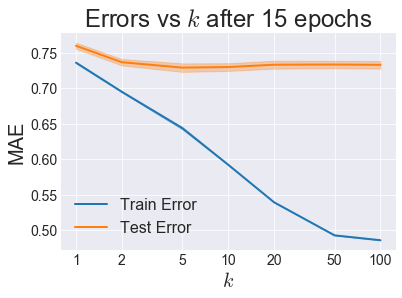This is the fouth post of a project on collaborative filtering based on the MovieLens 100K dataset. The remainder of this post is straight out of a Jupyter Notebook file you can download here. You can also see it here on GitHub.

Previously, I showed how to use similarity-based approaches that guess unknown user-movie-rating triplets by looking at either movies with a similar rating profile or users with a similar rating profile. These approaches leave a lot of data on the table though. Matrix factorization is a way to both take into account more data and perform some regularizing dimensionality reduction to help deal with the sparsity problem.

The basic idea is to organize the user-movie-rating triplets into a matrix with each row representing a user and each column representing a movie. We want to approximate this large matrix with a matrix multiplication of 2 smaller matrices. In the example below, each row of the "User Matrix" has 2 latent features of that user, and each column of the "Item Matrix" has 2 latent features of that item. The dot product of any user's latent features and item's latent features will give an estimate of the rating that user would give that movie.Image Source: https://medium.com/@connectwithghosh

There are many variations on this theme and multiple ways to perform this matrix factorization. The method I demonstrate here is called "Alternating Least Squares" method which was designed for the Netflix Prize and described in this paper. This method works iteratively, with 2 main steps per iteration:

1. Assume the User Matrix is fixed and solve for the Item Matrix
2. Assume the Item Matrix is fixed and solve for the User Matrix

In :
Expand Code

# 2. Load the Data¶

Let's load and examine the ratings data. If you're following along (i.e. actually running these notebooks) you'll need to make sure to run the first one to download the data before running this one.

In :
Expand Code
First 5:

userId movieId rating timestamp
214 259 255 4 1997-09-19 23:05:10
83965 259 286 4 1997-09-19 23:05:27
43027 259 298 4 1997-09-19 23:05:54
21396 259 185 4 1997-09-19 23:06:21
82655 259 173 4 1997-09-19 23:07:23
Last 5:

userId movieId rating timestamp
46773 729 689 4 1998-04-22 19:10:38
73008 729 313 3 1998-04-22 19:10:38
46574 729 328 3 1998-04-22 19:10:38
64312 729 748 4 1998-04-22 19:10:38
79208 729 272 4 1998-04-22 19:10:38

In :
Expand Code
In :
Expand Code

# 4. Determine how many epochs are necessary¶

In :
Expand Code
i_fold=0
i_fold=1
i_fold=2
i_fold=3
i_fold=4
CPU times: user 4min 46s, sys: 11.1 s, total: 4min 57s
Wall time: 2min 58s

In :
train_avg, train_std = train_errs.mean(axis=0), train_errs.std(axis=0)
test_avg, test_std = test_errs.mean(axis=0), test_errs.std(axis=0)
l, = plt.plot(np.arange(max_epochs), train_avg, label='Train Error')
plt.fill_between(np.arange(max_epochs), train_avg-train_std, train_avg+train_std,
color=l.get_color(), alpha=0.3)
l, = plt.plot(np.arange(max_epochs), test_avg, label='Test Error')
plt.fill_between(np.arange(max_epochs), test_avg-test_std, test_avg+test_std,
color=l.get_color(), alpha=0.3)
plt.legend()
plt.xlabel('Epoch')
plt.ylabel('MAE')
plt.title(r'Errors vs Epoch for $k=20$, $n_{ratings}=100K$')
plt.show()15 or 20 epochs seems like enough for Test Error to start plateauing.

# 5. Find Optimal $k$¶

In :
n_splits = 5
max_epochs = 15
kf = KFold(n_splits=n_splits, random_state=0, shuffle=True)
k_list = [1, 2, 5, 10, 20, 50, 100]
small_df = ratings_df.iloc[:100000]
train_errs = np.zeros((n_splits, len(k_list)))
test_errs = np.zeros((n_splits, len(k_list)))
for i_fold, (train_inds, test_inds) in enumerate(kf.split(small_df)):
print("i_fold={}: ".format(i_fold), end='')
train_df, test_df = small_df.iloc[train_inds], small_df.iloc[test_inds]
baseline_algo = DampedUserMovieBaselineModel(damping_factor=10)
for i_k, k in enumerate(k_list):
print("k={}, ".format(k), end='')
rec = ALSRecommender(k=k, baseline_algo=baseline_algo, verbose=False, max_epochs=max_epochs)
rec.fit(train_df)
preds = rec.predict(test_df[['userId', 'movieId']])
test_err = mean_absolute_error(preds, test_df['rating'])
test_errs[i_fold, i_k] = test_err
train_errs[i_fold, i_k] = rec.train_errors[-1]
print()

i_fold=0: k=1, k=2, k=5, k=10, k=20, k=50, k=100,
i_fold=1: k=1, k=2, k=5, k=10, k=20, k=50, k=100,
i_fold=2: k=1, k=2, k=5, k=10, k=20, k=50, k=100,
i_fold=3: k=1, k=2, k=5, k=10, k=20, k=50, k=100,
i_fold=4: k=1, k=2, k=5, k=10, k=20, k=50, k=100,

In :
train_avg, train_std = train_errs.mean(axis=0), train_errs.std(axis=0)
test_avg, test_std = test_errs.mean(axis=0), test_errs.std(axis=0)
l, = plt.semilogx(k_list, train_avg, label='Train Error')
plt.fill_between(k_list, train_avg-train_std, train_avg+train_std,
color=l.get_color(), alpha=0.3)
l, = plt.semilogx(k_list, test_avg, label='Test Error')
plt.fill_between(k_list, test_avg-test_std, test_avg+test_std,
color=l.get_color(), alpha=0.3)
plt.xticks(k_list, k_list)
plt.legend()
plt.xlabel(r'$k$')
plt.ylabel('MAE')
plt.title(r'Errors vs $k$ after {} epochs'.format(max_epochs))
plt.show()It looks like we have a Test Error minimum around $k=10$, although since $k=5$ is so close, I would go with that since it is likely to generalize better.

Check out the next notebook where I explore Stochastic Gradient Descent!